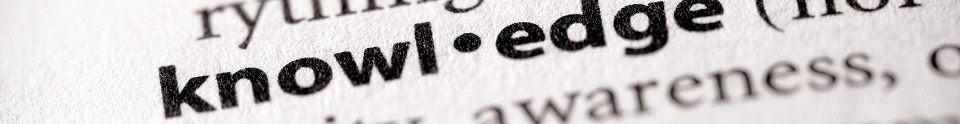# Jargon buster: 'Mass' to 'mnemonics'#### A | B–C | D–G | H–L | M | N–O | P | Q–R | S | T–Z

A | B | C | D | E | F | G | H | I | J | K | L | M | N | O | P | Q | R | S | T | U | V | W | X | Y | Z

### M

#### Measurements

Units of measure: An agreed quantity by which we measure things. So, metres, centimetres, litres, millilitres, grams and kilograms are all units of measure.

Metric measurement systems: Systems for measuring things in which the units of measure go up in 10s or multiples of 10 (100, 1000 and so on).

For example: Kilometres, metres, centimetres and millimetres are the metric system for measuring length and distance. There are:
10 millimetres in a centimetre.
100 centimetres in a metre.
1000 metres in a kilometre.

We now use metric systems for most of our measuring.

Imperial measurement systems: Systems for measuring things that were developed in England in the 19th century. Examples are pounds and ounces for measuring mass, and inches, yards and miles for measuring length and distance. We do not use them very much now, except miles for measuring distance and pints for volume.

Converting between units of measure: Changing a measurement from one unit (for example, metres) to another unit (for example, centimetres). So, the measurement 2 metres can be converted to 200 centimetres.

Length and distance are measured in kilometres, metres, centimetres and millimetres. There are:

10 millimetres in a centimetre.
100 centimetres in a metre.
1000 metres in a kilometre.

Mass is measured in grams and kilograms. There are:

1000 grams in a kilogram.

Volume is sometimes measured in litres and millilitres. There are:

1000 millilitres in a litre.

Converting between imperial and metric systems of measure: It can be useful to be able to convert between some common Imperial systems of measurement and the comparable metric system, for example, between miles and kilometres. To do this, you need to know the relationships between them. For instance, there are roughly:

1.6 kilometres in a mile.
0.6 miles in a kilometre.
2 pints in a litre.
0.5 litres in a pint.

Video: What are mass, volume and area?

#### Mental maths

The ability to calculate mentally, i.e. in your head without writing anything down. Learning things such as number bonds, number patterns, doubles and multiplication tables facts are important mental maths skills.

#### Missing number problems

A calculation where one of the numbers has been taken out. The missing number could be represented by a space, question mark or shape.

For example: 4 + ___ = 9. Children have to use related number facts to work out the answer. For instance, they may know that 9 – 4 = 5, and can therefore deduce that 4 + 5 = 9.

This type of question helps to prepare children for algebra, where unknown numbers are represented by letters.

#### Mixed number

A mixed number is another way of showing an improper fraction, by mixing a whole number and a proper fraction.

For example: 7/3 (an improper fraction) can also be represented as 2 ⅓ (a mixed number).

#### Modal verb

A type of auxiliary verb.

Modal verbs are: 'can', 'could', 'will', 'would', 'may', 'might', 'shall', 'should', 'must' and 'ought to'.

Modal verbs are used for expressing:

Possibility, ability or likelihood – 'can', 'could', 'may', 'might', 'should'.
Necessity – 'must', 'ought to', 'should'.
The future – 'shall', 'will', 'would'.

#### Morphology

A morpheme is a unit of meaning that cannot be divided further. Morphemes are the bits that make up words, such as read and ing in 'reading' or read and s in 'reads'. A word consists of one or more morphemes.

Some morphemes are words in their own right; others, such as many prefixes and suffixes, are not.

#### Mnemonics

Memory joggers such as a rhyme, a phrase or a shape. For example, seeing a dinosaur in the shape of a letter 'd' might help your child to associate the dinosaur with the letter 'd' and the 'd' sound.

Video: What are mnemonics?

#### A | B–C | D–G | H–L | M | N–O | P | Q–R | S | T–Z

A | B | C | D | E | F | G | H | I | J | K | L | M | N | O | P | Q | R | S | T | U | V | W | X | Y | Z